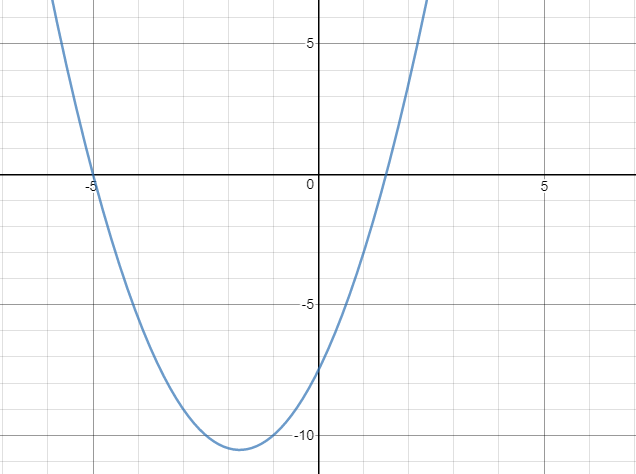# Exam-Style Questions on Quadratic Graph

## Problems on Quadratic Graph adapted from questions set in previous Mathematics exams.

### 1.

IB Analysis and Approaches

Consider the function $$f(x)=\frac{1}{2}\left(2x-3\right)\left(x+5\right)$$ for $$x \in \mathbb R$$. The following diagram shows part of the graph of $$f$$.For the graph of $$f$$

(a) find the coordinates of the x-intercepts.

(b) find the coordinates of the vertex.

The function $$f$$ can be written in the form $$f(x) = (x+h)^2 + k$$

(c) Write down the value of $$h$$ and the value of $$k$$.

### 2.

IB Analysis and Approaches

The graphs of the functions $$f(x)$$, a parabola, and $$g(x)$$, a straight line, meet at exactly one point.

$$f(x) = px^2 - px$$ $$g(x) = px-5$$

where $$x \in \mathbf R \text{ and } p \in \mathbf R$$

(a) Show that $$p = 5$$

The function $$f$$ can be expressed in the form $$f(x) = 5(x-m)(x-n) \text{, where } m,n \in \mathbf R$$

(b) Find the value of $$m$$ and the value of $$n$$.

The function $$f$$ can also be expressed in the form $$f(x) = 5(x-h)^2 + k, \text{ where } h,k \in \mathbf R$$

(c) Find the value of $$h$$ and the value of $$k$$.

(d) Hence find the values of $$x$$ where the graph of $$f$$ is both negative and decreasing.

If you would like space on the right of the question to write out the solution try this Thinning Feature. It will collapse the text into the left half of your screen but large diagrams will remain unchanged.

The exam-style questions appearing on this site are based on those set in previous examinations (or sample assessment papers for future examinations) by the major examination boards. The wording, diagrams and figures used in these questions have been changed from the originals so that students can have fresh, relevant problem solving practice even if they have previously worked through the related exam paper.

The solutions to the questions on this website are only available to those who have a Transum Subscription.

Exam-Style Questions Main Page

Search for exam-style questions containing a particular word or phrase:

To search the entire Transum website use the search box in the grey area below.